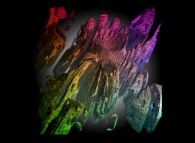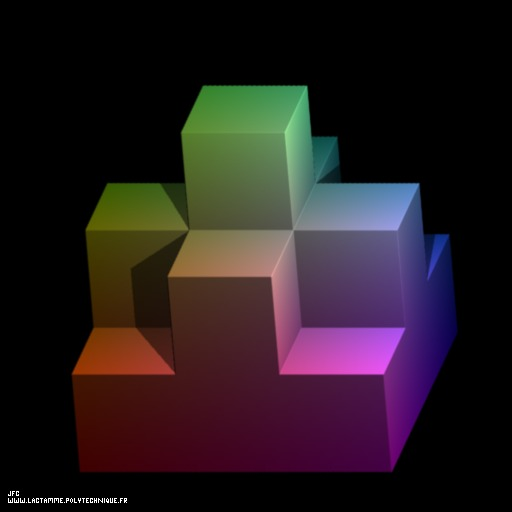An extended Menger sponge -iteration 1- [Une éponge de Menger généralisée -itération 1-].

See the first objects of this family (including this one):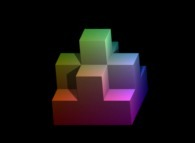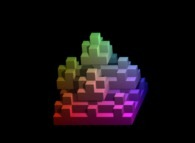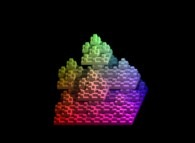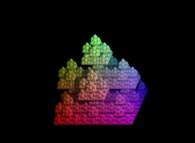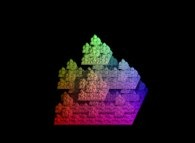```(SubdivisionRules=TTT FTF FFF  TTT TTT FTF  TTT FTF FFF)
```

Definition of the "standard" Menger sponge: A cube is cut into 3x3x3=27 identical smaller cubes. Then the 7 central subcubes (6 for each face and 1 at the center of the cube) are removed. At last this process is iterated recursively with the 27-7=20 remaining subcubes. The fractal dimension of the Menger sponge is equal to:
```                     log(20)
--------- = 2.726833027860842...
log(3)
```
The "standard" Menger sponge can be defined by means of subdivision rules. Here is the way how each of the 27 cubes of the "standard" Menger sponge at a given level is subdivided:
```
"standard"  Menger sponge
_____________________
/                     \

TTT       TFT       TTT
TFT       FFF       TFT
TTT       TFT       TTT

\_/

Sierpinski carpet
```
or again:
```                    TTT TFT TTT  TFT FFF TFT  TTT TFT TTT
```
where 'T' ('True') and 'F' ('False') means respectively "subdivide" and "do not subdivide". The rules are repeated at each level, but they can be changed periodically and for example:
```
TTT TFT TTT  TFT FFF TFT  TTT TFT TTT   FFF FTF FFF  FTF TTT FTF  FFF FTF FFF

\___________________________________/   \___________________________________/

"standard"  Menger sponge                      complement
```
alternates the "standard" Menger sponge and its complement. Obviously many other rules do exist as shown below...

Beside 'F' and 'T' some other possibilities exist: 'R' that means "subdivide" or "do not subdivide" Randomly with a given threshold between 0 and 1 (0.5 being the default value) and 'S' that means "Stop subdividing". Obviously 'F', 'T', 'R' and 'S' can be mixed at will...

Moreover an amazing cross-section can be made using the plane:
```                    2X - 2Y + 2Z - 1 = 0
```
the origin of the coordinates being at the center of the main cube and the axis being parallel to its sides.

This process can be generalized in many different ways and for example:
```                      3     3     3
2X  - 2Y  + 2Z  - 1 = 0
```
(the curved one) or again:
```                          1  2         1  2         1  2    2
(X - ---)  + (Y - ---)  + (Z - ---)  = R
2            2            2
```
(the spherical one).

The "standard" Menger sponge:

See some related pictures (possibly including this one):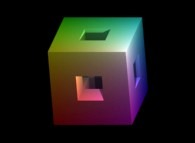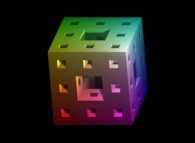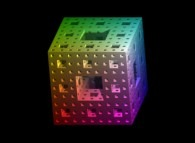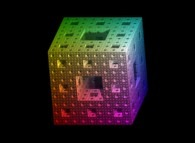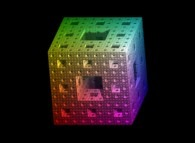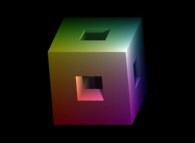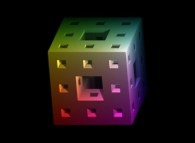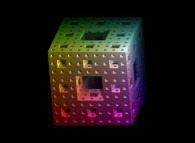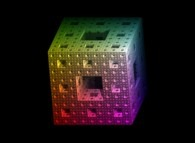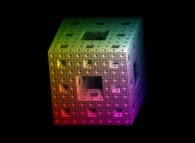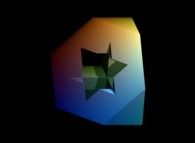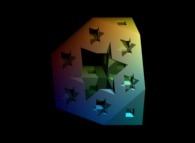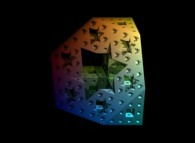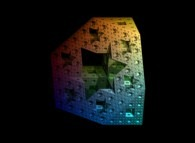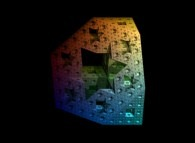==>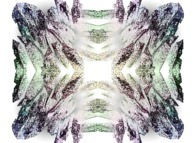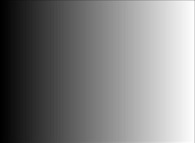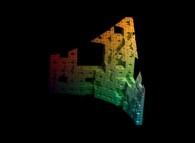==>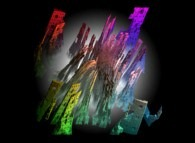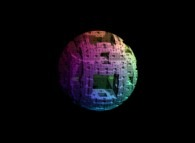==>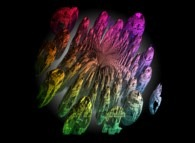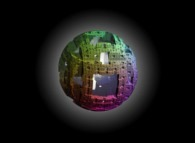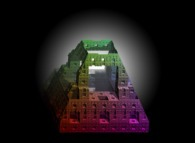==>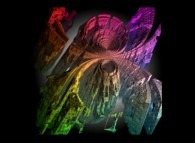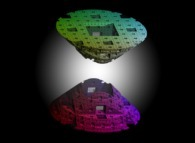==>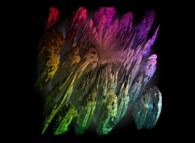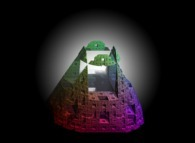==>